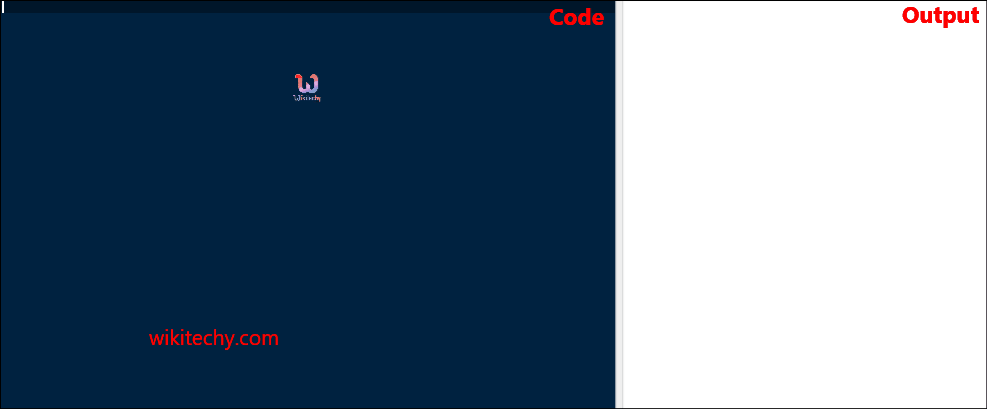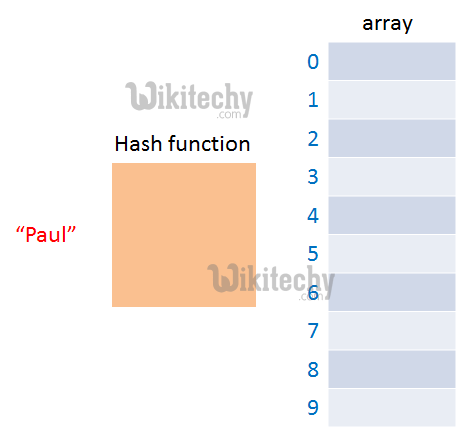C# array functions | C# array to string | C# Passing Array to Function - c# - c# tutorial - c# netLearn c# - c# tutorial - C# Array Functions - c# examples - c# programs

How to passing array to function in C# ?

• In C#, to reuse the array logic, we can create function.
• To pass array to function in C#, we need to provide only array name.Passing Array Function

C# Passing Array to Function Example: print array elements

• Let's see an example of C# function which prints the array elements.

C# Passing Array to Function Example: Print minimum number

• Let's see an example of C# array which prints minimum number in an array using function.

C# Passing Array to Function Example: Print maximum number

• Let's see an example of C# array which prints maximum number in an array using function.